# TlH (thallium hydride) Spin-Orbit Coupling¶

This tutorial consists of several steps:

• TlH spin-orbit fragment analysis
• Separate calculations for Tl and H
• Visualization of the energy diagram
• Visualization of spinors
• Calculate the atomization energy

## Step 1: Prepare molecule¶

First create a TlH (thallium hydride) molecule with a bond length of 1.87 Angstrom (the experimental bond length):

Open ADFinput and draw a TlH molecule.
Select the Tl and H atoms
Use the slider to set the distance between the atoms 1.87 Angstrom## Step 2: Set calculation options¶

Next we will set up the calculation. The following details need to be set:

Clear the selection (click in empty drawing space)

Select the GGA → BP86 as XC functional
Select the ‘Spin-Orbit’ Relativity option
Select the basis set ‘TZ2P’
Set Frozen core to ‘None’

The Main panel will now look like: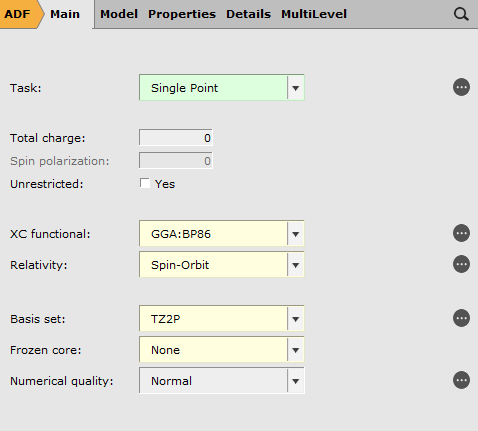We are going to perform a fragment analysis as a trick to get a diagram that makes it very easy to compare scalar and spin-orbit relativistic results.

Fragment calculations are based on regions, which are just collections of atoms. So we start by making a region:

Select both atoms
Use the panel bar Model → Regions command
Click the ‘+’ button to add a new region
Change the name of the new region (Region 1) to TlH_SR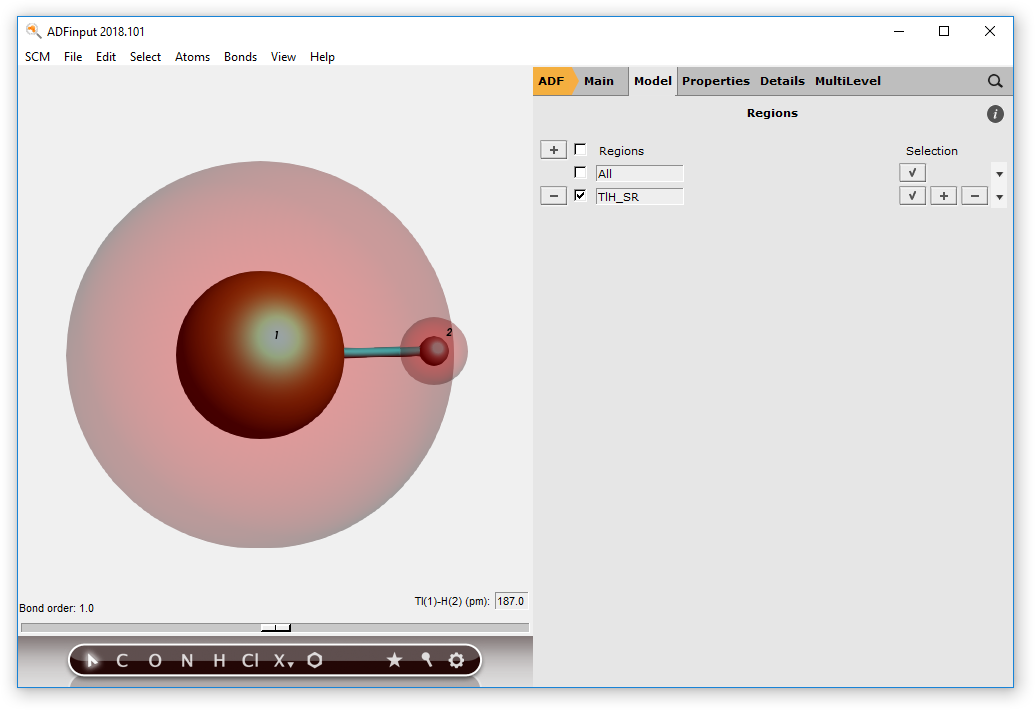You have now defined a region containing all atoms, with name TlH_SR.

Use the panel bar Multilevel → Fragments menu command
Click the ‘Use fragments’ check box## Step 3: Run your calculation¶

Use the File → Save menu command
Enter the name ‘TlH_SO’ in the ‘Filename’ field
Click ‘Save’

Now you have saved your current options and molecule information.

As we have set up a fragment calculation, also the .adf and .run files for the fragment have been saved. Lets study what options are used for the fragment in ADFinput:

Make sure the ‘Fragments’ panel is still the current panel
Click on the ‘Open’ button (the dot) for the TlH_SR fragment

A new ADFinput window will also appear with the name ‘ADFinput: TlH_SO.TlH_SR.adf’. This is the name of the molecule, a dot, and the name of the fragment. The fragment should have the ‘Scalar’ relativistic option selected, as that is required when the results will be used as a fragment. The other options are identical to what you set for the main molecule.

Select File → Close

We are now ready to run the calculation:

Select File → Run

Now two calculations will run: first the building fragment (using the scalar relativistic option), and next the version including spin-orbit coupling. You will see the two logfiles. Wait until both calculations have finished:

Wait until ADFtail shows ‘Job ... has finished’ as last line
Select File → Close
Repeat for the second ADFtail, thus closing both logfiles

## Step 4: Results of the calculation¶

### TlH energy diagram¶

To see the effect of the spin-orbit coupling we will first look at the energy level diagram:

SCM → Levels
Select View → Labels → Show

Press and hold the Right mouse button on the stack name ‘TlH_SO’,

Click on ‘Zoom HOMO-9 .. LUMO+9’.
Next try to zoom using a drag with the right mouse button, or using the scroll wheel.
Do this such that only levels between -0.1 and -0.7 eV are shown.
You can move the levels vertically by dragging with the left mouse button.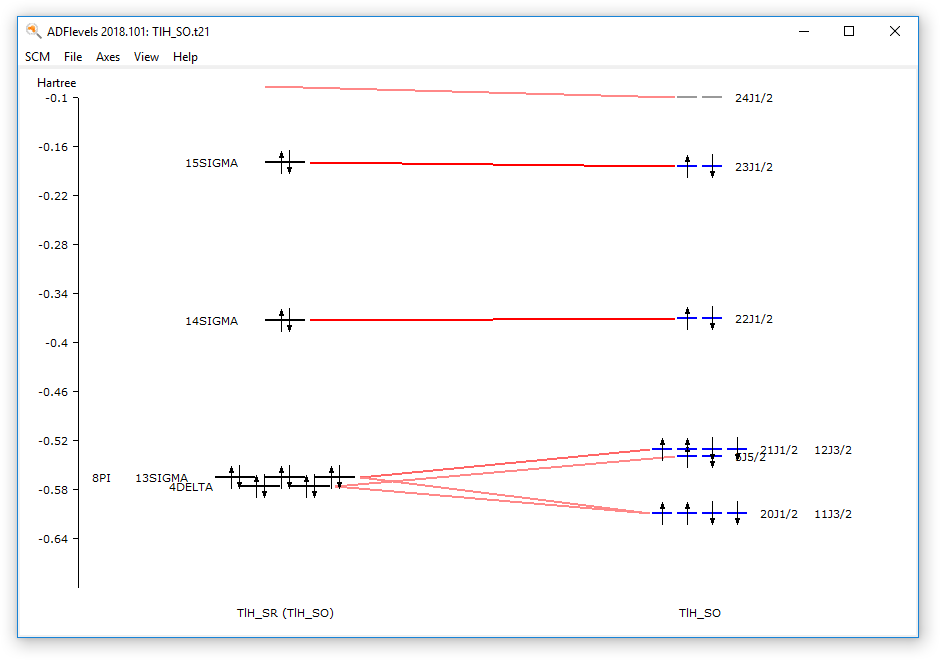You can see that the spin-orbit coupling is important to split energy levels.

Especially for the Tl core levels the spin-orbit coupling is more important than the ligand field splitting. Compare the 8pi, 13sigma, 4delta orbitals (close to 5d atomic Tl orbitals) with the 11j3/2, 20j1/2 spinors (close to 5d3/2 atomic Tl spinors) and 5j5/2, 12j3/2, and 21j1/2 spinors (close to 5d5/2 atomic Tl spinors).

If you press and hold the right mouse button on one of the levels, you can select a spinor. That spinor will be shown. You can also show all spinors (in the case of a degenerate level) at once.

The energy diagram of the scalar relativistic fragment calculation shows the atomic contributions to the scalar relativistic levels.

Select the TlH_SO.TlH_SR job (the scalar relativistic fragment)
Use the SCM → levels command
Select View → Labels → Show
Press and hold the Right mouse button on the stack name ‘TlH_SO.TlH_SR’,
Click on ‘Zoom HOMO-4 .. LUMO+4’.
Next zoom and move the levels using a right mouse drag and or scroll wheel.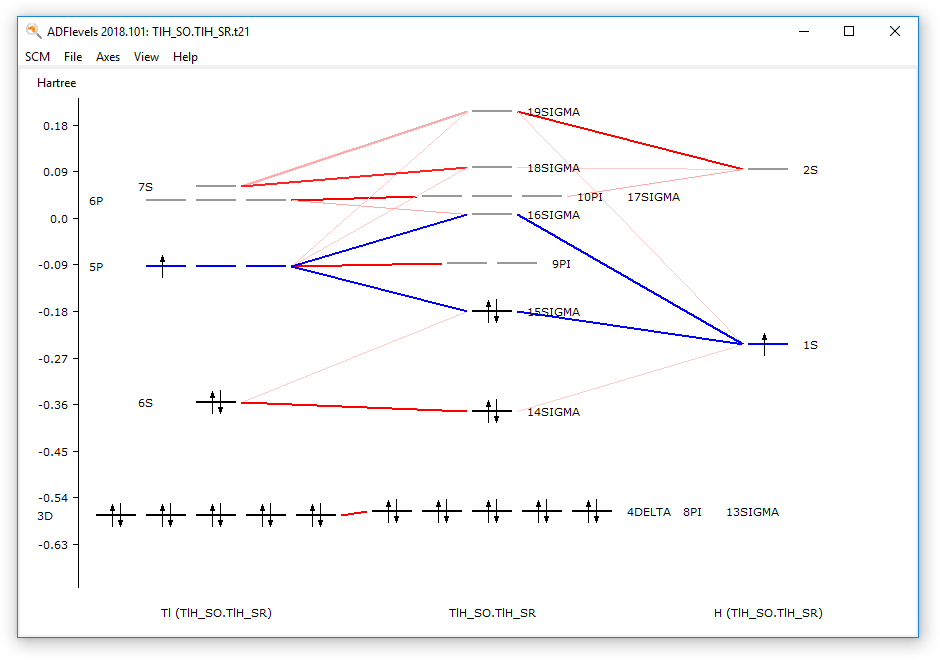### Visualization of spinors¶

Visualization of spinors is conceptually more difficult than visualization of orbitals.

A spinor $$\Psi$$ is a two-component complex wave function, which can be described with four real functions $$\phi$$: real part $$\alpha$$ $$\phi_\alpha^R$$ , imaginary part $$\alpha$$ $$\phi_\alpha^I$$ , real part $$\beta$$ $$\phi_\beta^R$$ , imaginary part $$\beta$$ $$\phi_\beta^I$$:

$\Psi = \binom{\phi_\alpha^R + i \phi_\alpha^I}{\phi_\beta^R + i \phi_\beta^I}$

The density $$\rho$$ is:

$\rho = \Psi^\dagger \Psi$

The spin magnetization density $$m$$ is:

$m = \Psi^\dagger \sigma \Psi$

where $$\sigma$$ is the vector of the Pauli spin matrices $$\sigma_x$$, $$\sigma_y$$, and $$\sigma_z$$. A spinor is fully determined by the spin magnetization density and a phase factor $$e^{i \theta}$$, which both are functions of spatial coordinates.

In ADFview one can visualize the (square root of the) density and spin magnetization density, however, the phase factor $$e^{i \theta}$$ is summarized only with a plus or minus sign.

For this tutorial we have a small molecule, and a fine grid is chosen for better visualization.

Select SCM → View
Rotate the molecule, such that one can see both atoms.
Select Fields → Grid → Fine
Select Add → Spinor: Spin Magnetization Density

In the new control line at the bottom, use the field select pull-down menu and
Select Orbitals (occupied) ....
Select the J1/2:1 number 22 spinor.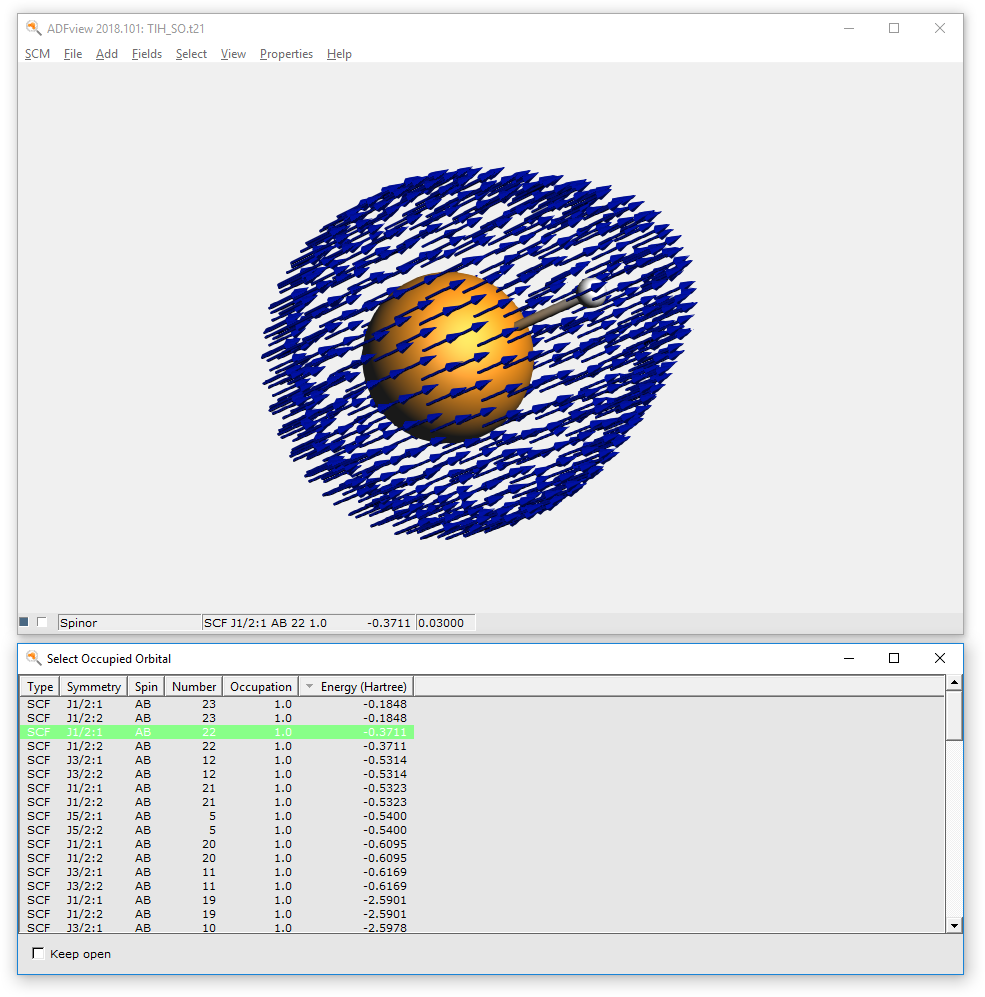The arrows in this picture are in the direction of the spin magnetization density m. All arrows are approximately in the same direction, which means that this spinor is an eigenfunction of spin in this direction of the arrows. In fact this 22 j1/2 spinor is almost a pure $$\alpha$$ orbital. The arrows are drawn starting from points in space where the square root of the density is 0.03. The color of the arrows is red or blue by default, indicating minus or plus for the phase factor $$e^{i \theta}$$ .

The (square root of the) density and the approximate phase vector $$e^{i \theta}$$ can also be viewed separately:

Select Add → ‘Isosurface: With Phase
Select Orbitals (occupied)...
Select the SCF_J1/2:1 number 18 spinor.
Hide the spinor (uncheck the check box at the left of the Spinor label)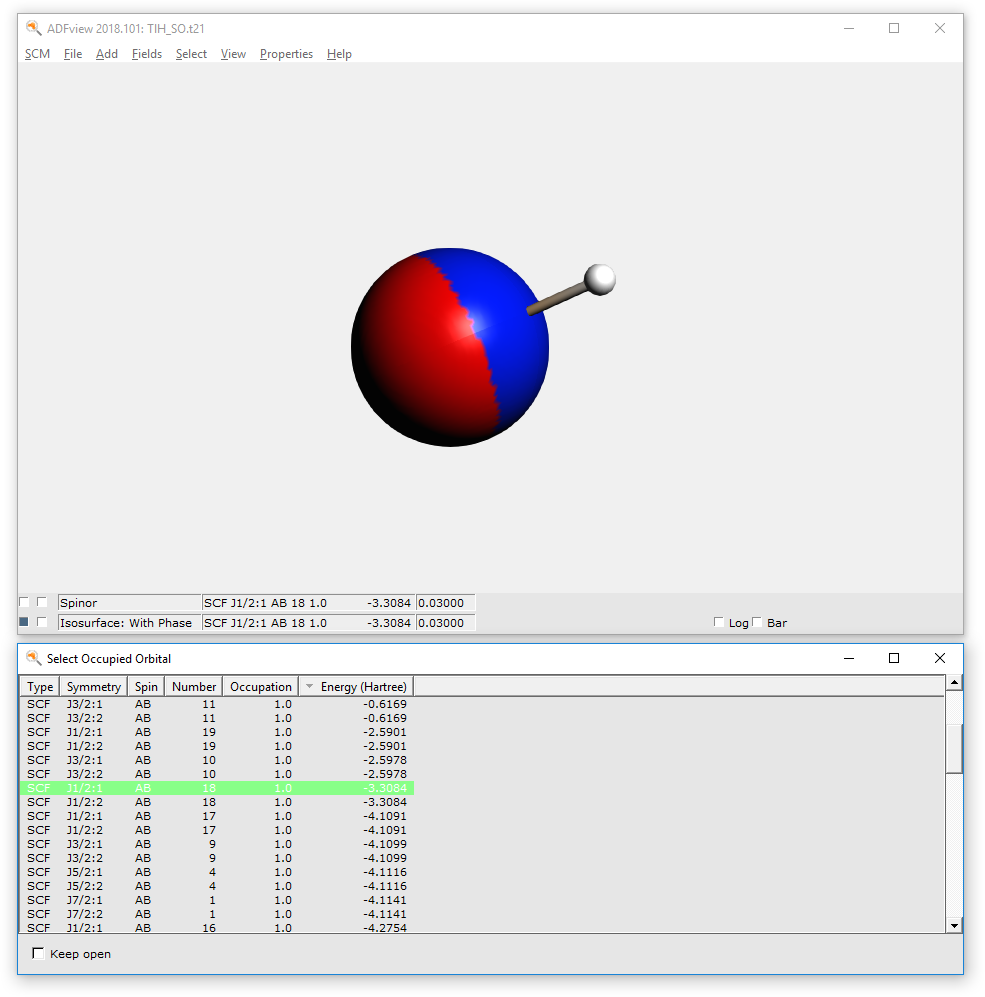This spinor 18j1/2 is almost a pure 5p1/2 Tl spinor. A p1/2 atomic orbital has a spherical atomic density, but a spin magnetization density which is not the same in each point in space.

In the control line with ‘Spinor’, press on the pull-down menu and
Select Orbitals (occupied)
Select the SCF_J1/2:1 number 18 spinor
Show the ‘Spinor’ (check the left check box for the spinor line)
Hide the ‘isosurface with phase’ (uncheck the left check box for the isosurface with phase line)
Hide the atoms: View → Molecule → Sticks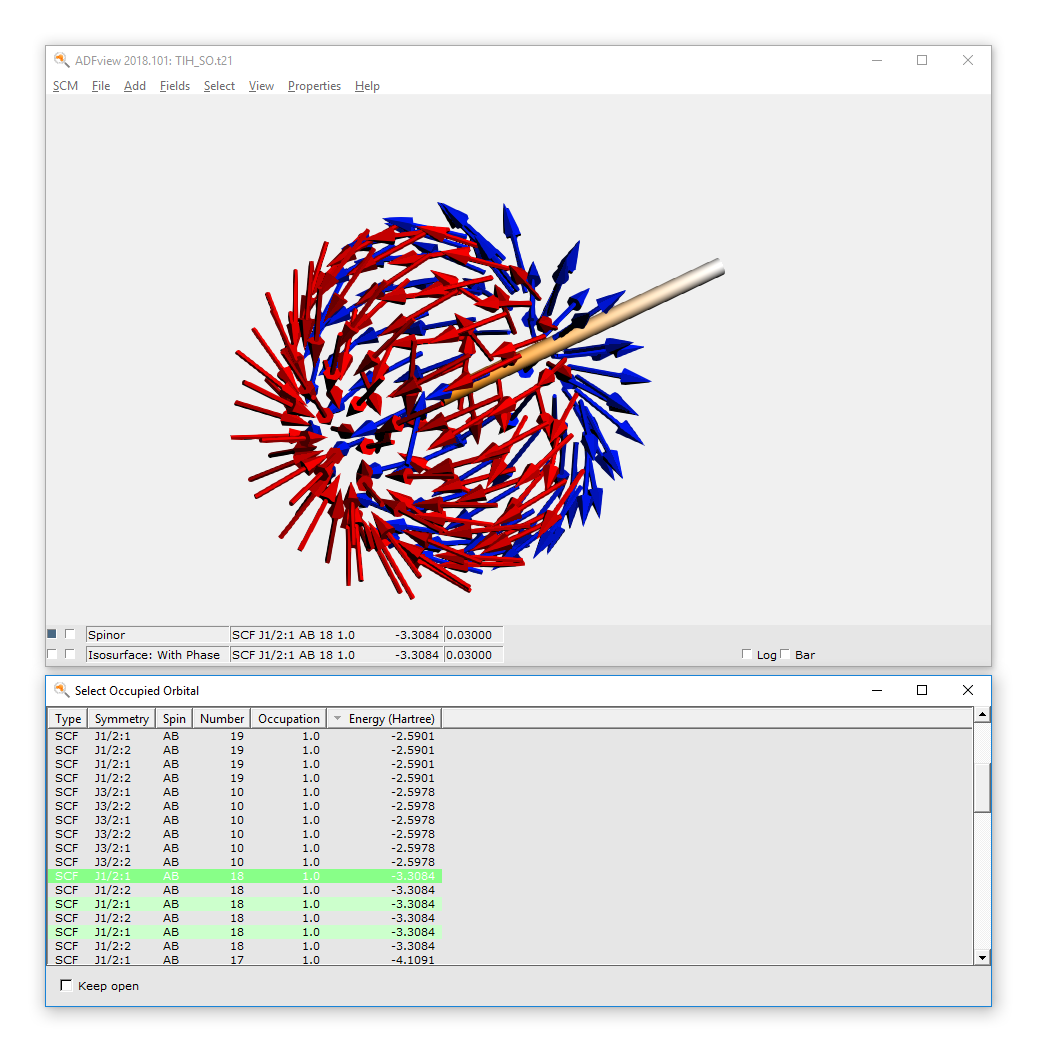## Step 5: Calculate the atomization energy including spin-orbit coupling¶

The calculation of the atomization energy is not a simple problem in DFT. Spin-orbit coupling is an extra complication. In this paragraph a way is presented how to calculate the atomization energy using spin-polarized calculations in the non-collinear approximation.

If you wish, you can skip the rest of this tutorial.

### The Tl atom¶

To calculate an atomization energy we need to calculate the atoms also including spin-orbit coupling. The easiest way is to start with the TlH_SO.adf file and change this to an atomic file.

Since the Tl atom is an open shell atom for an (accurate) atomization energy we need to do an unrestricted calculation. The best theoretical method is the non-collinear method. Note that the ‘Spin polarization’ field is not used in the non-collinear method.

Delete the H atom (select it and press the backspace key)
Use the panel bar Multilevel → Fragments command
Uncheck the ‘Use fragments’ option
Use the panel bar Model → Regions command
Remove the TlH_SR region (click on the - button in front of it)
Select ‘Main’ panel
Check the ‘Unrestricted:’ box

Select the Relativity panel (search for relativity)
Select ‘NonCollinear’ from the ‘Spin polarization’ options
Select File → Save As
Enter the name ‘Tl_SO’ in the ‘FileName’ field
Click on ‘Save’
Click OK to acknowledge the warning about fractional occupation numbersNow we want to actually perform the calculation for the Tl atom

Run the calculation: File → Run
Wait until ADFtail shows ‘Job ... has finished’ as last line
In the window showing the logfile (the ADFtail window Tl_SO.logfile):
Select File → Close

### The H atom¶

Basically we can follow the same steps as for the Tl atom, but in this case we will start with Tl_SO.adf file and change this.

Select the ‘Main’ panel
Select the Tl atom
Use the Atoms → Change Atom Type → H
Select File → Save As...
Enter the name ‘H_SO’ in the ‘Filename’ field
Click on ‘Save’
Select File → Run
Wait until ADFtail shows ‘Job ... has finished’ as last line
In the window showing the logfile (the ADFtail window H_SO.logfile):
Select File → Close

### TlH atomization energy¶

The atomization energy including spin-orbit coupling is a combination of several terms.

Select SCM → Logfile
Write down the value of the bonding energy printed at the end of the calculation
in the ADFtail window. (should be around -1038.62 eV)
Select File → Open
Select the file ‘TlH_SO.TlH_SR.logfile’
Write down the value of the bonding energy printed at the end of the calculation
in the ADFtail window. (should be around -3.84 eV)
Select File → Open
Select the file ‘Tl_SO.logfile’
Write down the value of the bonding energy printed at the end of the calculation
in the ADFtail window. (should be around -1039.32 eV)
Select File → Open
Select the file ‘H_SO.logfile’
Write down the value of the bonding energy printed at the end of the calculation
in the ADFtail window. (should be around -0.95 eV)

The atomization energy including spin-orbit coupling is in this case, the bond energy printed in the TlH_SO.logfile plus the the bond energy printed in the TlH_SO.TlH_SR.logfile minus the bond energy printed in the Tl_SO.logfile minus the the bond energy printed in the H_SO.logfile. (approximately -1038.62 - 3.84 + 1039.32 + 0.95 = -2.19 eV, experimental number is close to -2.06 eV.)